# Polynomial of best approximation

Jump to: navigation, search

A polynomial furnishing the best approximation of a function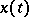in some metric, relative to all polynomials constructed from a given (finite) system of functions. Ifis a normed linear function space (such as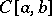or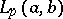,), and if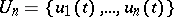is a system of linearly independent functions in, then for anythe (generalized) polynomial of best approximation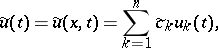(*)

defined by the relation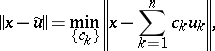exists. The polynomial of best approximation is unique for allifis a space with a strictly convex norm (i.e. if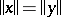and, then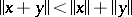). This is the case for,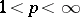. In, which has a norm that is not strictly convex, the polynomial of best approximation for anyis unique if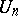is a Chebyshev system on, i.e. if each polynomialhas at most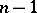zeros on. In particular, one has uniqueness in the case of the (usual) algebraic polynomials in, and also for the trigonometric polynomials in the space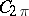of continuous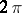-periodic functions on the real line, with the uniform metric. If the polynomial of best approximation exists and is unique for any, it is a continuous function of.

Necessary and sufficient conditions for a polynomial to be a best approximation in the spacesandare known. For example, one has Chebyshev's theorem: Ifis a Chebyshev system, then the polynomial (*) is a polynomial of best approximation for a function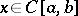in the metric ofif and only if there exists a system ofpoints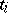,, at which the difference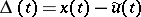assumes values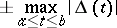and, moreover,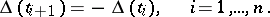The polynomial (*) is a polynomial of best approximation for a function,, in the metric of that space, if and only if for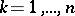,In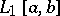, the conditions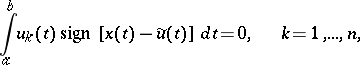are sufficient for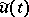to be a polynomial of best approximation for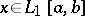, and if the measure of the set of all points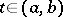at which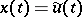is zero, they are also necessary; see also Markov criterion.

There exist algorithms for the approximate construction of polynomials of best uniform approximation (see e.g. , ).